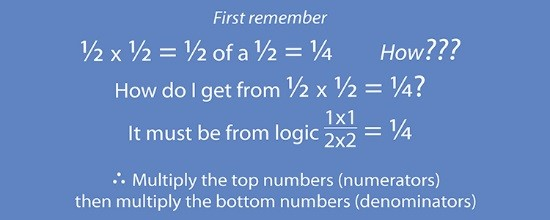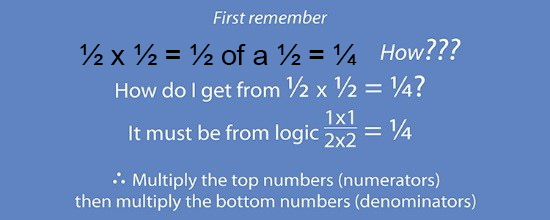# Multiplication Fractions = Think 1/2

Whenever you see the word fractions think of 1/2The fractured (fraction) rock was split in half (1/2)

To remember how to calculate fractions always think of a 1/2 and work it out from there:From this, you can use logic and ask yourself how do you get from 1/2 of a 1/2 to equal a 1/4

It must be: (1times1)/(2times2)=1/4 (It couldn’t be anything else)

So use this logic for all other multiplication of fractions.

Example 1

Work out  3/4\ times1/3Therefore 3/4\ times1/3=(3\times1)/(4times3)=3/12\ or\ 1/4

Example 2

What is  1/2\times 1/3Therefore 1/2\ times1/3=(1times1)/(2times3)=1/6

Example 3

Work out 1\3/4\times2\1/2

This means work out  7/4\times5/2Therefore 7/4\times5/2=35/8\ or\ 4\3/8

NOTE:

Do this on any multiplication of a fraction and you will always get the correct answer.